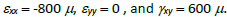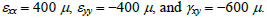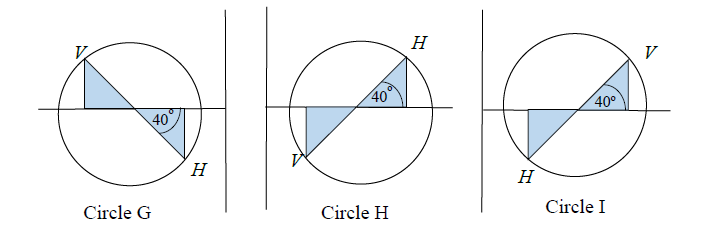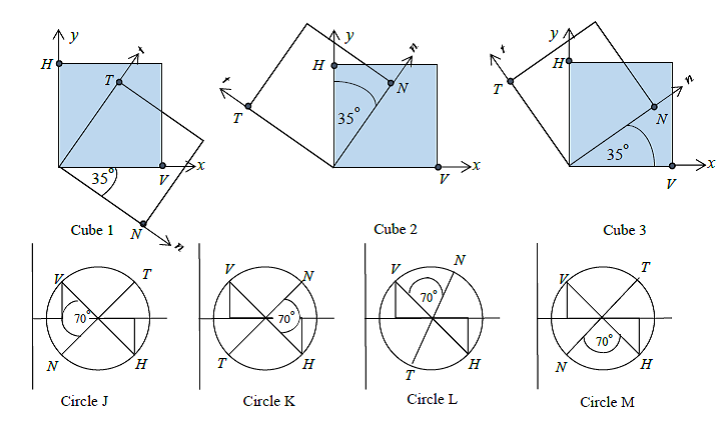9.1 Strain Transformation by Mohr Circle

1. The state of strainis associated with the Mohr's
2. The state of strainis associated with the Mohr's
3. The state of strainis associated with the Mohr's
4.The principal angle 1 calculated from circle G is
5. The principal angle 1 calculated from circle H is
6. The principal angle 1 calculated from circle I is
7.Cube 1 is associated with Mohr’s
8. Cube 2 is associated with Mohr’s
9. Cube 3 is associated with Mohr’s
10. Circle G is in plain strain with  principal strains  1 and 2 as 1200  and  200 micro, respectively. The maximum shear strain is